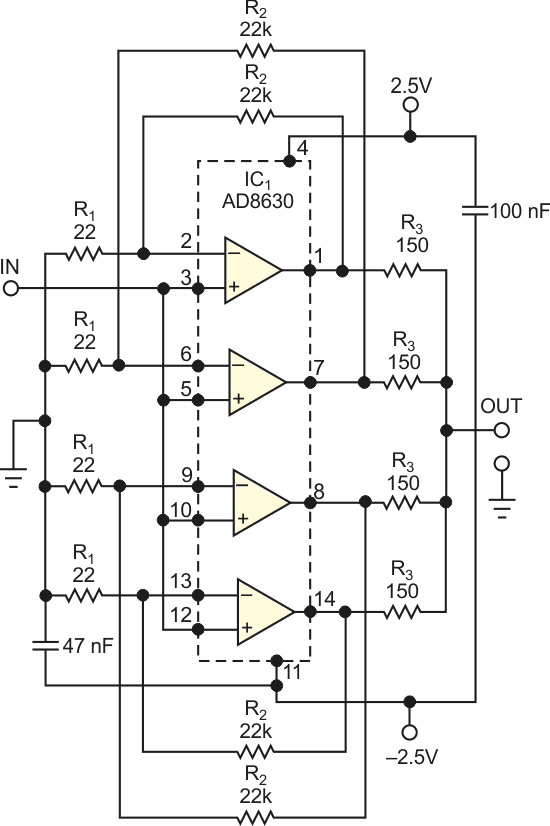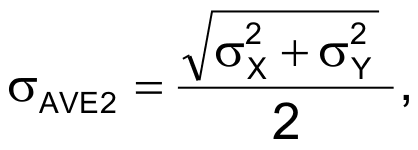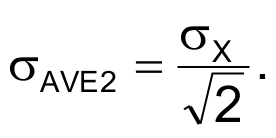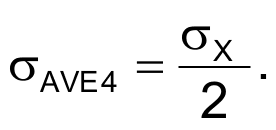# Paralleling decreases autozero-amplifier noise by a factor of two

## Analog Devices AD8630

Autozero amplifiers have almost zero drift and input-offset values of 1 to 20 µV. You can compensate for the initial voltage offset of an auto-zero amp in sensitive circuits, such as dc amplifiers and integrators, requiring the processing of voltages of 10 µV to 1 mV. Total compensation down to an offset of 0 V, however, is an illusion because residual low-frequency output noise is still present in any autozero amp.The Analog Devices AD8628 autozero amp has a low-frequency-noise value of 0.5 µV p-p at 0.1 to 10 Hz. If your application requires zero drift and low output noise, you can use the circuit in Figure 1. A quad autozero amp develops a gain of almost 1000. The resistor network comprising the R3 resistors averages the output signals of these amplifiers to create the final output voltage.Figure 1. Use this circuit when your application requires zero drift and low output noise.

The quad autozero amps are the four sections of IC1, an Analog Devices AD8630. Quad integrated resistors having one common lead can substitute for the four R3 resistors. The R1 and R2 resistors should be high-quality, precision, film devices with 0.5% or less tolerance. The tolerance of the R3 resistors should not exceed 1%. The basis for decreasing the circuit’s noise at the output in comparison with a single amplifier of IC1 is the principle of averaging the signals containing the same deterministic component of random noise. If you assume that the amplifiers of IC1 represent independent or uncorrelated noise sources that obey the gaussian distribution, then the standard deviation of the average of noise outputs of these sections is:where σX and σY are the standard deviations of noise signals at outputs of the single respective amplifiers. If σX = σY – an assumption that you can make without hesitation because the op amps reside in one chip – then:If you average four amplifiers, you obtain:If the value of output resistance of the circuit, which is about R3/4 ≈ 38 Ω, is too high for your application, place a voltage follower between the output terminal and the next stage.

## Materials on the topic

EDN

You may have to register before you can post comments and get full access to forum.
 User Name Remember Me? Password